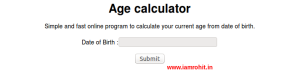# Calculate Age from Date of Birth in PHP

Today I am going to show you how easily you can calculate the age of the user from date of birth. Some web based applications are needed to show the age of the user. In that case, you need to calculate the user’s age from date of birth. Here I have written quick php function to calculate age from date of birth in php using php date_diff() function. With the help of this php function you can easily calculate the difference between two dates.You can add below function in your php library and use any where to calculate the age of the user by their date of birth.

```<?php function getAge(\$dob) { \$today = date("Y-m-d"); \$diff = date_diff(date_create(\$dob), date_create(\$today)); return \$diff->format('%yYears, %mMonths, %dDays'); }   echo getAge('19-10-1988'); ?>```

Output: 27Years, 10Months, 19Days

Have a look, I have created simple online program to calculate user’s current age from date of birth by using above php function.

Posted in PHP